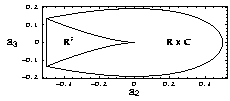# John Jones - PublicationsOutside Links ASU Main ASU site School of Mathematical and Statistical Sciences The School/Department main site

## Papers

1. The L-functions and modular forms database, with J. Cremona, A. Sutherland, and J. Voight, Notices Amer. Math. Soc., to appear.
2. Computing septic number fields, with E. Driver, J. Number Theory, 202, 426-429 (2019). (preprint)
3. Mixed degree number field computations, with D. Roberts, Ramanujan J., 47:1 47-66 (2018). (arxiv version)
4. Artin L-functions of small conductor, with D. Roberts, Research in Number Theory, 3:16 (2017).
5. A database of number fields, with D. Roberts, LMS J. Comput. Math., 17, 595-618 (2014). (arxiv version)
6. The tame-wild principle for discriminant relations for number fields, with D. Roberts, Algebra and Number Theory, 8 no. 3, 609-645 (2014).
7. Minimal solvable nonic fields, LMS J. Comput. Math., 16, 130-138 (2013).
8. Number fields with solvable Galois groups and small Galois root discriminants, with R. Wallington, Math. Comp., 81, 555-567 (2012).
9. Looking at Groups, Res. J. Math. Technol., 1, 111-120 (2012). Also published in Elec. J. Math. Technol., 6, 1933-2823 (2012).
10. Wild ramification bounds and simple group Galois extensions ramified only at 2, Proc. Amer. Math. Soc., 139, 807-821 (2011).
11. Minimum discriminants of imprimitive decic fields, with E. Driver, Exp. Math., 19, 475-479 (2010).
12. Number fields unramified away from 2, J. Number Theory, 130, 1282-1291 (2010).
13. Inertia subgroups for octic 2-adic fields, Int. J. Pure Appl. Math., 61, 301-314 (2010).
14. A targeted Martinet search, with E. Driver, Math. Comp., 78, 1109-1117 (2009).
15. $\mathbf{Z}$-cyclic whist tournaments for $q^2$ players, with P. Leonard, J. Combin. Math. Combin. Comput., 66, 215-223 (2008).
16. Octic 2-adic fields, with D. Roberts, J. Number Theory, 128, 1410-1429 (2008).
17. Number fields ramified at one prime, with D. Roberts, in ANTS VIII, Springer Lecture Notes in Computer Science, 5011, 226-239 (2008).
18. Galois number fields with small root discriminant, with D. Roberts, J. Number Theory, 122, 379-407 (2007).
19. A database of local fields, with D. Roberts, J. Symbolic Comput., 41, 80-97 (2006).
20. Nonic 3-adic fields, with D. Roberts, in ANTS VI, Springer Lecture Notes in Computer Science, 3076, 293-308 (2004).
A revision correcting an error in the published version.
21. Septic fields with discriminant $\pm 2^a 3^b$, with D. Roberts, Math Comp., 72, 1975-1985, (2003).
22. Sextic number fields with discriminant $(-1)^j 2^a 3^b$ , with D. Roberts, in Number Theory: Fifth Conference of the Canadian Number Theory Association, CRM Proceedings and Lecture Notes, 19, American Math. Soc., 141-172 (1999).
23. Teaching Number Theory with CAS or Java, with J. Holt, Proceedings for the 11th Annual International Conference on Technology in Collegiate Mathematics, 178-182, (1999).
24. Timing analysis of targeted Hunter searches, with D. Roberts, in ANTS III, Springer Lecture Notes in Computer Science, 1423, 412-423 (1998).
25. Discovering Number Theory, with J. Holt. Proceedings for the 10th Annual International Conference on Technology in Collegiate Mathematics, 211-215, (1998).
26. Zero free regions for a rational function with applications, with B. Welfert, Advances in Computational Mathematics, 3, 265-289 (1995).
27. Plater's $p$-adic orthogonality relation for abelian varieties, Houston J. Math., 21, 261-282 (1995).
28. Iwasawa $L$-functions of elliptic curves with additive reduction, J. Number Theory, 51, 103-117 (1995).
29. On the equation $x^4+mx^2y^2+y^4=z^2$, with A. Bremner, J. Number Theory, 50, 286-298 (1995).
30. Iwasawa L-functions and the mysterious $\cal L$-invariant, in p-adic Monodromy and the Birch and Swinnerton-Dyer Conjecture, AMS Contemporary Math Series 165, 63-70 (1994).
31. On the local norm map for abelian varieties with good ordinary reduction, J. Algebra 138, 420-423 (1991).
32. A comparison of Selmer groups, Manuscripta Math. 68, 391-398 (1990).
33. $p$-adic heights for semi-stable abelian varieties, Compositio Math. 73, 31-56 (1990).
34. Iwasawa $L$-functions for multiplicative abelian varieties, Duke Math. J. 59, 399-420 (1989).

## Books

1. Discovering Number Theory, with J. Holt, W.H. Freeman and Company, New York (2001).
2. Instructor's Manual for Discovering Number Theory cd-rom, with J. Holt, W.H. Freeman and Company, New York (2001).
3. Arithmetic Geometry: Conference on Arithmetic Geometry with an Emphasis on Iwasawa Theory, March 15-18, 1993 at Arizona State University, coeditor with N. Childress, AMS Contemporary Math. Series, 174, (1994).
Last Update: June 15, 2021
 Physical Sciences, A-Wing Apply to ASU: undergraduate | graduate Last Modified: June 15, 2021